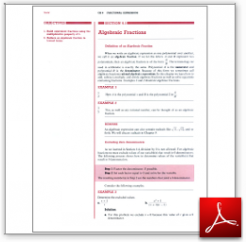Algebra Math Book - Introductory Algebra Thank you for your continual support.

 The power of mathematics rests in the logic of thinking.ID: Sec6-1
Description: Definition of Algebraic Fraction-Zero Denominators-Building Equivalent Fractions-Reducing
Price: 1.99

# Algebra Math book - Introductory Algebra - Chapter 6 - Section 1 - Algebraic Fractions

## Section 6.1 - Objectives

1.  Build equivalent fractions using the multiplicative property of 1.

2.  Reduce an algebraic fraction to the lowest terms.

Navigate to
Next Section
or Chapter 6 Details or Other Chapters

This section of my algebra math book, Introductory Algebra, also includes in the download:

• Cover Sheet